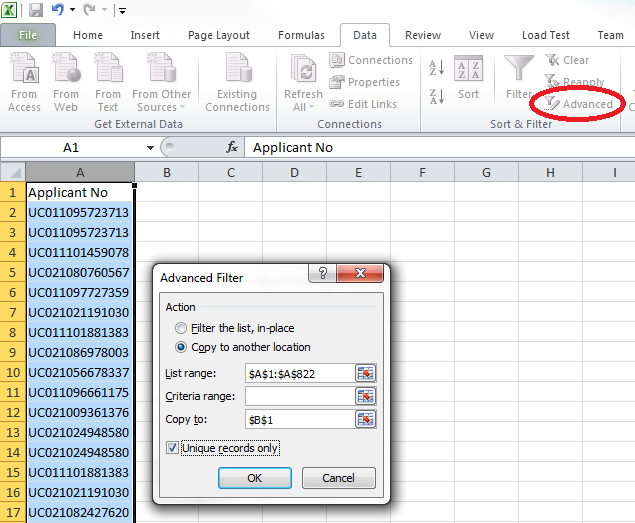# Select unique values in Microsoft Excel column

Thought I'd put a quick note here, I tried a fair few solutions that didn't work and then found this hidden away in a forum:

Quick Count
```=INT(SUMPRODUCT((A3:A1000<>"")/COUNTIF(A3:A1000,A3:A1000&"")))
```

This returns the number of unique values in the range A3 to A1000 and excludes the blank/empty cells.

Display all Unique
Found this note on one of Microsoft Help sites:
Office 2010:
1. Select the data range to do this to.
2. Click on the "Data" tab.
4. Tick the "Unique records only" box.A few more from Microsoft Office Help
```/* Count the number of unique number values in cells A2:A10, but do not count blank cells or text values (4) */
=SUM(IF(FREQUENCY(A2:A10,A2:A10)>0,1))

/* Count the number of unique text and number values in cells B2:B10 (which must not contain blank cells) (7) */
=SUM(IF(FREQUENCY(MATCH(B2:B10,B2:B10,0),MATCH(B2:B10,B2:B10,0))>0,1))

/* Count the number of unique text and number values in cells A2:A10 , but do not count blank cells or text values (6) */
=SUM(IF(FREQUENCY(IF(LEN(A2:A10)>0,MATCH(A2:A10,A2:A10,0),""), IF(LEN(A2:A10)>0,MATCH(A2:A10,A2:A10,0),""))>0,1))
```

### Accreditation### Donate & Support

If you like my content, and would like to support this sharing site, feel free to donate using a method below:

Paypal:Bitcoin:3QnhmaBX7LQSRsC9hh6Je9rGQKEGNQNfPb